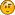Hello Guest it is August 03, 2020, 06:08:29 AM

###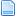Author Topic: Speed up problem  (Read 1321 times)

0 Members and 1 Guest are viewing this topic.

####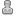AleksanderA2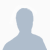•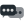2##### Speed up problem
« on: March 25, 2015, 04:21:43 PM »
Hi

I'm trying to print something with my 3d clay printer driven by Geckodrive G540.
For code generator I use Slic3r
Till now everything was ok. You see the clay is pushed by compressed air and for printing vases this goes ok without having to interupt the clay flow.
However for non printing parameters I tried to speed up the printing proces so the flow would be interrupted.

Image:The guys at Slic3r forum told me, the code is ok, but there is no speed up at those points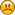Can anyone look at the code and help?

Thank you!

AleX

Code: [Select]
`; generated by Slic3r 1.2.6 on 2015-03-25 at 14:00:41; external perimeters extrusion width = 3.00mm; perimeters extrusion width = 3.36mm; infill extrusion width = 3.36mm; solid infill extrusion width = 3.36mm; top infill extrusion width = 3.36mmM104 P200 ; set temperatureG28 ; home all axesG1 Z5 F5000 ; lift nozzleM109 P200 ; wait for temperature to be reachedG21 ; set units to millimetersG90 ; use absolute coordinatesM106 P89.25G1 Z1.250 F12000.000G1 A-2.00000 F2400.00000G1 X82.265 Y70.236 F12000.000G1 A0.00000 F2400.00000M204 S100G1 X83.039 Y69.359 A0.46162 F4200.000G1 X83.765 Y68.632 A0.86693..................G1 X86.390 Y109.814 A4424.63265G1 X86.333 Y107.344 A4426.78462G1 X86.333 Y99.459 A4433.65486G1 A4431.65486 F2400.00000M107M104 S0 ; turn off temperatureG28 X0  ; home X axisM84     ; disable motors; filament used = 4433.7mm (31.3cm3); avoid_crossing_perimeters = 0; bed_shape = 0x0,200x0,200x200,0x200; bed_temperature = 0; before_layer_gcode = ; bridge_acceleration = 0; bridge_fan_speed = 100; brim_width = 0; complete_objects = 0; cooling = 0; default_acceleration = 100; disable_fan_first_layers = 0; duplicate_distance = 6; end_gcode = M104 S0 ; turn off temperature\nG28 X0  ; home X axis\nM84     ; disable motors\n; extruder_clearance_height = 20; extruder_clearance_radius = 20; extruder_offset = 0x0; extrusion_axis = E; extrusion_multiplier = 1; fan_always_on = 1; fan_below_layer_time = 70; filament_diameter = 3; first_layer_acceleration = 0; first_layer_bed_temperature = 0; first_layer_extrusion_width = 200%; first_layer_speed = 70; first_layer_temperature = 200; gcode_arcs = 0; gcode_comments = 0; gcode_flavor = mach3; infill_acceleration = 0; infill_first = 0; layer_gcode = ; max_fan_speed = 100; min_fan_speed = 35; min_print_speed = 70; min_skirt_length = 0; notes = ; nozzle_diameter = 3; only_retract_when_crossing_perimeters = 1; ooze_prevention = 0; output_filename_format = [input_filename_base].gcode; perimeter_acceleration = 0; post_process = ; pressure_advance = 0; resolution = 0; retract_before_travel = 2; retract_layer_change = 0; retract_length = 2; retract_length_toolchange = 10; retract_lift = 0; retract_restart_extra = 0; retract_restart_extra_toolchange = 0; retract_speed = 40; skirt_distance = 6; skirt_height = 1; skirts = 1; slowdown_below_layer_time = 0; spiral_vase = 0; standby_temperature_delta = -5; start_gcode = G28 ; home all axes\nG1 Z5 F5000 ; lift nozzle\n; temperature = 200; threads = 2; toolchange_gcode = ; travel_speed = 200; use_firmware_retraction = 0; use_relative_e_distances = 0; use_volumetric_e = 0; vibration_limit = 0; wipe = 0; z_offset = 0; dont_support_bridges = 1; extrusion_width = 0; first_layer_height = 50%; infill_only_where_needed = 0; interface_shells = 0; layer_height = 2.5; raft_layers = 0; seam_position = aligned; support_material = 0; support_material_angle = 0; support_material_contact_distance = 0.2; support_material_enforce_layers = 0; support_material_extruder = 1; support_material_extrusion_width = 0; support_material_interface_extruder = 1; support_material_interface_layers = 3; support_material_interface_spacing = 0; support_material_interface_speed = 100%; support_material_pattern = pillars; support_material_spacing = 2.5; support_material_speed = 70; support_material_threshold = 0; xy_size_compensation = 0; bottom_solid_layers = 0; bridge_flow_ratio = 1; bridge_speed = 60; external_fill_pattern = rectilinear; external_perimeter_extrusion_width = 0; external_perimeter_speed = 50%; external_perimeters_first = 0; extra_perimeters = 1; fill_angle = 45; fill_density = 0%; fill_pattern = honeycomb; gap_fill_speed = 20; infill_every_layers = 1; infill_extruder = 1; infill_extrusion_width = 0; infill_overlap = 15%; infill_speed = 80; overhangs = 1; perimeter_extruder = 1; perimeter_extrusion_width = 0; perimeter_speed = 70; perimeters = 1; small_perimeter_speed = 70; solid_infill_below_area = 70; solid_infill_every_layers = 0; solid_infill_extruder = 1; solid_infill_extrusion_width = 0; solid_infill_speed = 20; thin_walls = 1; top_infill_extrusion_width = 0; top_solid_infill_speed = 15; top_solid_layers = 0`

####AleksanderA2•2##### Re: Speed up problem
« Reply #1 on: March 26, 2015, 08:14:30 AM »
Anybody?